# ISEE Middle Level Quantitative : Outcomes

## Example Questions

1 2 4 Next →

### Example Question #31 : Data Analysis And Probability

A pair of fair six-sided dice are thrown, and the sum of the numbers facing upward is noted. What is the probability that the sum will be 7 or less?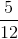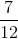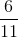Explanation:

There are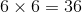possible rolls of two fair six-sided dice, each of which will come up with equal probability. The set of rolls is shown below, with the ways to roll a 7 or less indicated.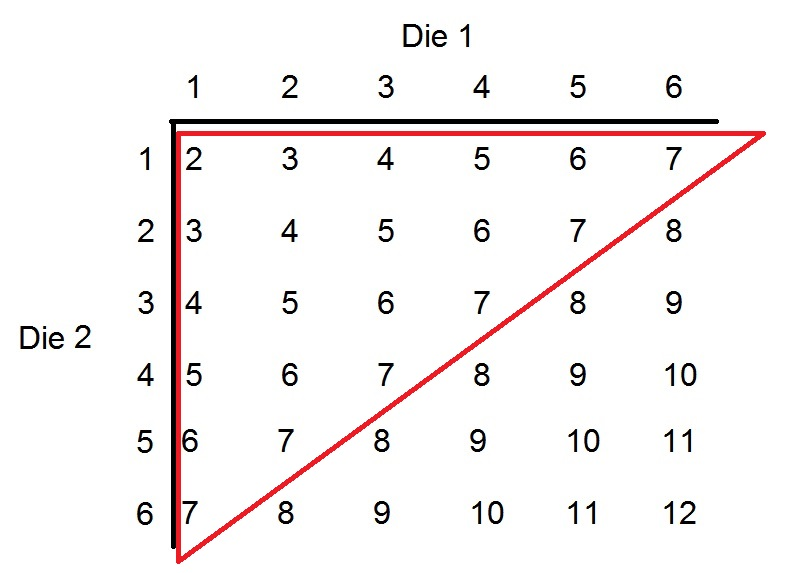There are 21 ways out of 36 to roll a sum of 7 or less, making the probability of this outcome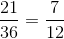.

### Example Question #31 : Probability

Each of a set of balls is marked with a number; one ball is marked with a "1", two balls are marked with a "2". and so forth up to ten balls with a "10". The odd-numbered balls are placed in Box 1; the rest, in Box 2. The boxes contain no other balls.

Which is the greater quantity?

(a) The probability that a ball randomly drawn from Box 1 will be marked "1".

(b) The probability that a ball randomly drawn from Box 2 will be marked "2".

It is impossible to determine which is greater from the information given

(b) is the greater quantity

(a) is the greater quantity

(a) and (b) are equal

(b) is the greater quantity

Explanation:

Box 1 contains the odd-numbered balls, of which there are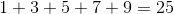.

One of these balls is marked with a "1", making the probability of drawing this ball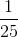.

Box 2 contains the even-numbered balls, of which there are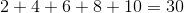.

Two of these balls are marked with a "2", making the probability of drawing such a ball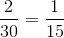.

Between two fractions with the same numerator, the one with the lesser denominator is the greater, so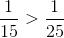, and the greater probability is that of drawing a "2" from Box 2.

### Example Question #31 : Data Analysis And Probability

Each of a set of balls is marked with a number; one ball is marked with a "1", two balls are marked with a "2". and so forth up to ten balls with a "10". The odd-numbered balls are placed in Box 1; the rest, in Box 2.

Which is the greater quantity?

(a) The probability that a ball randomly drawn from Box 1 will be marked "9".

(b) The probability that a ball randomly drawn from Box 2 will be marked "10".

It is impossible to determine which is greater from the information given

(a) is the greater quantity

(b) is the greater quantity

(a) and (b) are equal

(a) is the greater quantity

Explanation:

Box 1 contains the odd-numbered balls, of which there are.

Nine of these balls are marked with a "9", making the probability of drawing one of these balls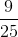.

Box 2 contains the even-numbered balls, of which there are.

Ten of these balls are marked with a "10", making the probability of drawing such a ball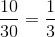.

We can compare these fractions by expressing both with a common denominator.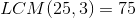, so we can rewrite these probabilities as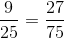and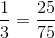.

The probability that a ball randomly drawn from Box 1 will be marked "9" is the greater.

### Example Question #31 : Probability

A large box contains some balls, each marked with a whole number from "1" to "10". Each number is represented by one red ball. In addition, each prime number is represented by one green ball, and each composite number is represented by one blue ball. A yellow ball, which is not marked with a number, is also included.

Give the probability that a randomly-drawn ball will be blue.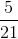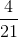Explanation:

Each number will be represented by one red ball, so there will be ten red balls in the box.

The prime numbers - the numbers that have only 1 and themselves as factors - are 2, 3, 5, and 7, so there will be four green balls.

The composite numbers - the numbers that have more than two factors - are 4, 6, 8, 9, and 10, so there will be five blue balls.

Note that 1 is neither prime nor composite.

Including the unmarked yellow ball, the total number of balls is, five of which are blue, so the probability of drawing a blue ball at random is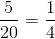.

### Example Question #32 : Outcomes

John has a standard deck of 53 cards, which includes the joker. Mary has two decks of 52 cards, shuffled together without their jokers. John and Mary each select a card at random from their decks. Which is the greater quantity?

(a) The probability that John will draw a diamond

(b) The probability that Mary will draw a diamond

(b) is the greater quantity

(a) is the greater quantity

(a) and (b) are equal

It is impossible to determine which is greater from the information given

(b) is the greater quantity

Explanation:

One fourth of the cards in a standard deck of 52, not including the joker, will be diamonds; if two standard decks are shuffled together, then one fourth of those cards will be diamonds. The probability that Mary will draw a diamond is therefore.

However, when the joker is added to John's deck, there are more cards, but just as many diamonds. Therefore, the probability of John drawing a diamond must decrease to slightly less than. This gives Mary a greater probability of drawing a diamond.

### Example Question #31 : Data Analysis And Probability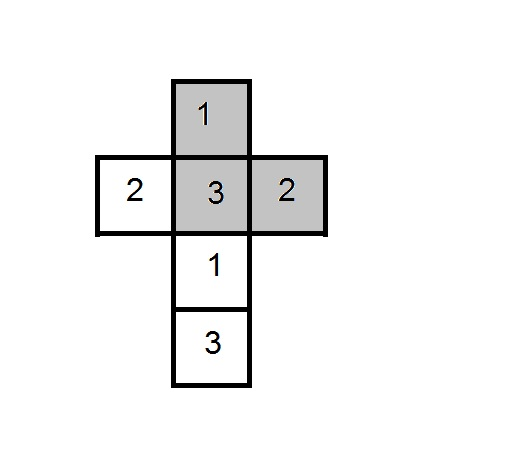The six faces of a die are shown in the fold-out diagram above; this die and an identical one are tossed.

Which is the greater quantity?

(a) The probability that the dice land with sides of the same color facing upward

(b) The probability that the dice land with sides of different colors facing upward

(a) and (b) are equal

(a) is the greater quantity

(b) is the greater quantity

It is impossible to determine which is greater from the information given

(a) and (b) are equal

Explanation:

As seen from the table below, out of the thirty-six equally probable rolls, eighteen result in the same color facing upward on both dice (indicated by an "S"); eighteen result in different colors facing upward (indicated by a "D").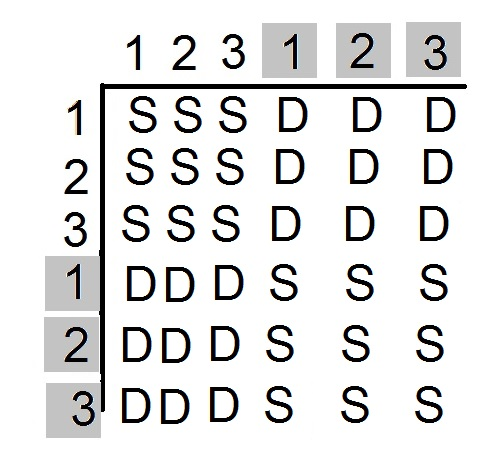The events occur with the same frequency, so they also occur with the same probability.

### Example Question #33 : Data Analysis And ProbabilityThe six faces of a fair die are shown in the fold-out diagram above; this die and an identical one are tossed.

Which is the greater quantity?

(a) The probability that the sum of the numbers facing upward is even

(b) The probability that the sum of the numbers facing upward is odd

(a) is the greater quantity

It is impossible to determine which is greater from the information given

(b) is the greater quantity

(a) and (b) are equal

(a) is the greater quantity

Explanation:

As seen from the table below, out of the thirty-six equally probable rolls, twenty result in an even sum (these are set apart with red squares), and sixteen result in an odd sum.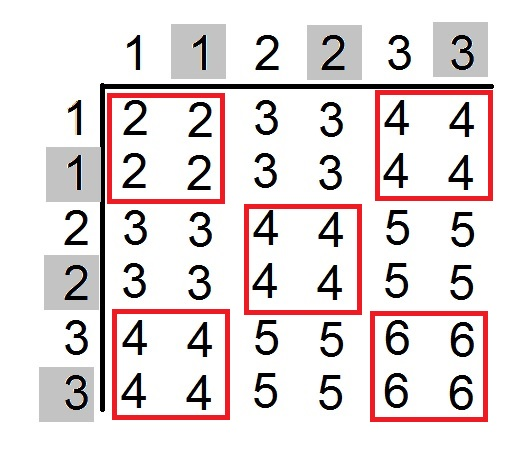Since even sums happen with greater frequency, the probability of an even sum is greater.

### Example Question #31 : Probability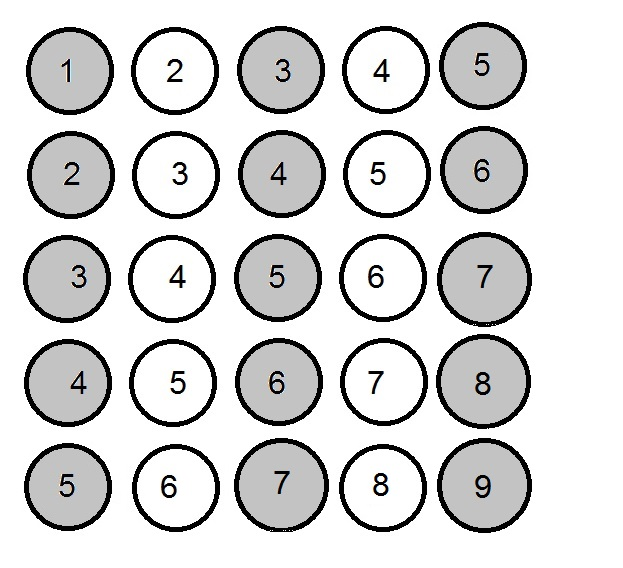The above diagram shows a set of balls. The odd-numbered balls are placed in Box A; the even-numbered balls, in Box B. Which is the greater quantity?

(a) The probability that a ball randomly drawn from Box A will be white.

(b) The probability that a ball randomly drawn from Box B will be white.

(b) is the greater quantity

It is impossible to determine which is greater from the information given

(a) is the greater quantity

(a) and (b) are equal

(b) is the greater quantity

Explanation:

The diagram below shows how the balls are separated.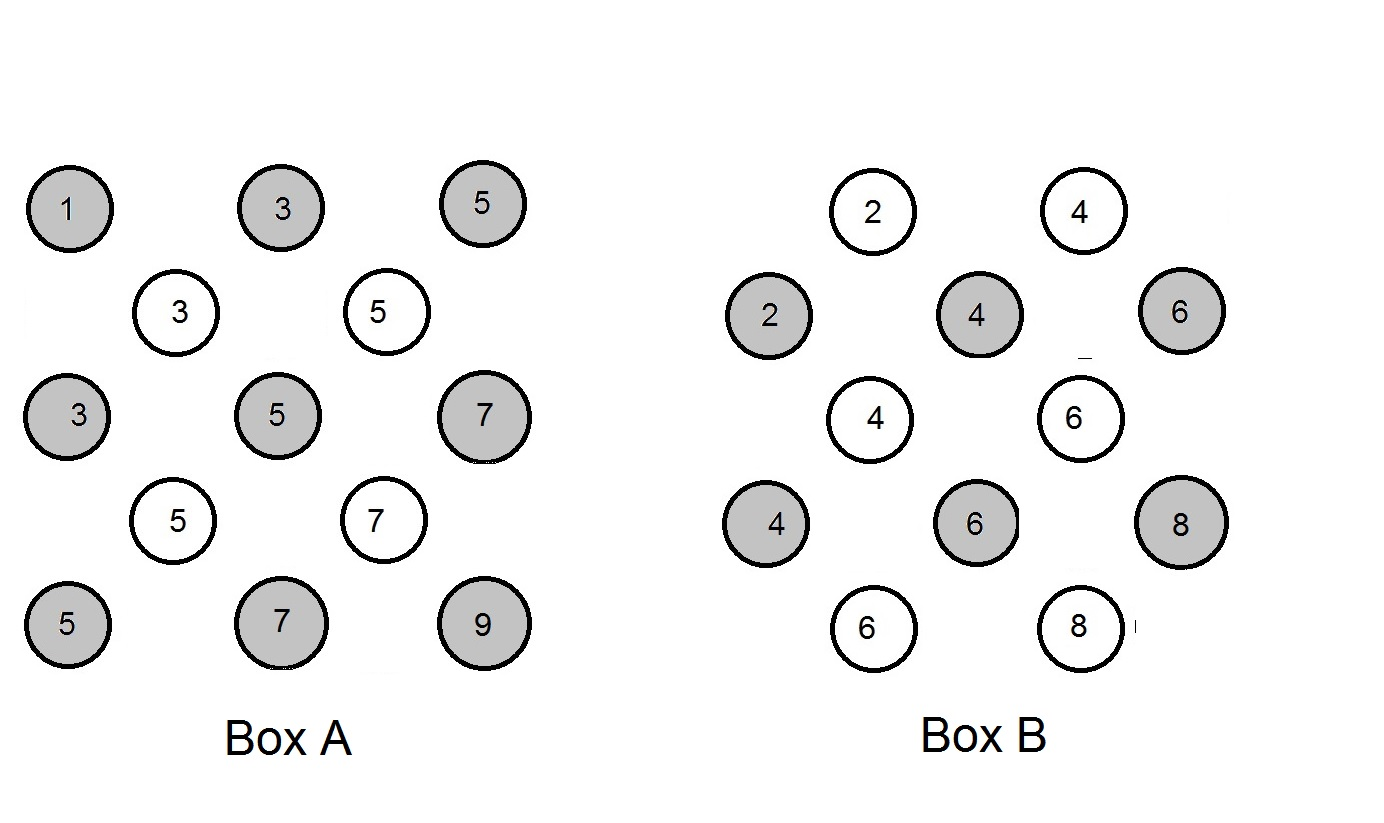Box A contains 13 balls, of which 4 are white; the probability of a random draw from this box resulting in the draw of a white ball is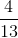Box B contains 12 balls, of which 6 are white;  the probability of a random draw from this box resulting in the draw of a white ball is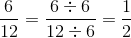.

Rewriting the probabilities in terms of a common denominator: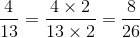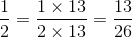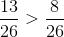, so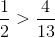, making the draw of a white ball more likely from Box B.

### Example Question #32 : Probability

Two fair six-sided dice are thrown. What is the probability that the product is greater than or equal to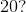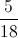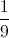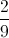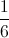Explanation:

The rolls that yield a product greater than or equal to 20 are: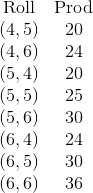These are 8 out of 36 rolls, so the probability of getting one of them is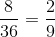1 2 4 Next →

### All ISEE Middle Level Quantitative Resources